# Computational study for reliability improvement of a circuit board

## Abstract

### Background

An electronic device consists of electronic components attached on a circuit board. Reliability of such a device is limited to fatigue properties of the components as well as of the board. Printed circuit board (PCB) consists of conducting traces and vertical interconnect access (via) out of copper embedded in a composite material. Usually the composite material is fiber reinforced laminate out of glass fibers and polyimid matrix. Different reasons play a role by choosing the components of the laminate for the board, one of them is its structural strength and fatigue properties. An improvement of board’s lifetime can be proposed by using computational mechanics.

### Methods

In this work we present the theory and computation of a simplified one layer circuit board conducting electrical signals along its copper via, producing heat that leads to thermal stresses.

### Results

Such stresses are high enough to perform a plastic deformation. Although the plastic deformation is small, subsequent use of the electronic device causes accumulating plastic deformation, which ends the lifetime effected by a fatigue failure in the copper via.

### Conclusion

Computer simulations provide a convenient method for understanding the nature of this phenomenon as well as predicting the lifetime. We present a coupled and monolithic way for solving the multiphysics problem of this electro-thermo-mechanical system, numerically, by using finite element method in space and finite difference method in time.

## Background

Considering electronic devices, the fatigue failure occurs more frequently under cyclic loadings. In a daily use of an electronic device, we switch some transistors on and off such that heat is produced on the component and traces as well as vias (wires conducting electric signals). This heat increases the temperature of the circuit board. As a consequence, copper and the composite material try to expand differently—regarding their coefficients of thermal expansion—so-called thermal stresses occur. Unfortunately, such stresses are higher than the yield stress such that plastic deformation is induced. Since the produced heat escapes the device by an active or passive cooling, the electronic device tries to shrink or expand to its original shape. Due to the plastic deformation, this shape change generates stresses again. Hence a cyclic loading implies a plastic deformation in each cycle. The plastic deformation is irreversible and in each cycle the amount of plastic deformation accumulates. Sooner or later, there appear cracks caused by fatigue. In order to prevent these cracks, we may try to match the constants of thermal expansion of wire and composite material. Therefore, a possible improvement of fatigue properties in a circuit board relies on the choice of the composite material. In this study we investigate a non-conventional composite material and its effect to the reliability of the circuit board by using computation of thermo-electro-mechanical simulations.

Reliability tests of PCBs are performed in the design process. In order to accelerate the tests, electronic devices are placed in an oven and temperature in the oven is changed periodically by a given frequency and amplitude. Since the board is thin and metal components have a high thermal conductivity, a nearly homogeneous temperature distribution occurs. There is a significant amount of know-how for thermal reliability tests and manufacturers are using their own calibrated tests, i.e., choice of frequency and amplitude. In order to obtain results as quick as possible, the oven achieves more than 100 K in less than a minute, which is not only technologically challenging; but also costly. Another method is much more easier and is sometimes called an active reliability test. An electric potential difference is applied such that an electric current produces JOULE’s heat leading to the temperature change. According to the free or forced convection, the necessary temperature differences in similar frequencies can be achieved. There are still some drawbacks and a lack of a comprehensive analysis of active tests. Computational methods can be fruitful for getting a better understanding and suggesting newer methods or design amendments. In this work we present the method of solving a coupled thermo-electro-mechanical system with open-source packages developed under the FEniCS project, see (FEniCS project 2017; Alnaes and Mardal 2012). Coupled and nonlinear partial differential equations can be solved monolithically by using research codes, for example FEniCS. Commercial programs are not capable to perform such tasks, at least at the time when this work was established. In order to demonstrate the strength of such computation, we perform an active reliability test for different laminate materials and compare them. We deliver the codes applied on a single thru hole via on PCB with different materials used for the board. Different materials as well as geometries can easily be applied by using the code in (Abali 2011) under the GNU Public license (GNU Public 2017).

## Methods

We follow closely (Abali 2016, Sect. 3.4) and outline herein the theory as well as the method of computation very briefly. The objective is to simulate an unpopulated circuit board consisting of one thru hole via. Copper via is a conductor and is embedded in the composite, which is an insulator. In order to set the ideas, consider Fig. 1. The board is clamped on the four chamfer faces. As in a real experiment, we can set the electric potential, ϕ in V(olt), on the ends of the via at front and back faces (on yz-planes) of the board. The electric potential difference creates an electric field, E i in V/m(eter), leading to an electric current,in A(mpere)/m2, measured on the material frame. In other words, this current is the effective motion of charges with respect to the continuum body. Independently, the body can have a motion such as deformation, too. A deformation of the material is observed with respect to the laboratory frame. The electric current in the laboratory frame is given by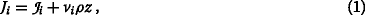where ρ denotes the mass density in k(ilo)g(ram)/m3, z the specific charge in C(oulomb)/kg, and v i the velocity of the continuum body as rate of displacement, v i =ui∙. Strictly speaking, the formulation is in the reference placement; however, by assuming small deformations we refrain from distinguishing between reference and current placement. Since the formulation is in the reference placement, the time rate (·) is simply the partial time derivative. We search for the displacement, u i , effected by the electric potential set on each end of the via. Concretely, we set one end zero (grounded); on the other end we apply a harmonic excitation with a relatively low frequency, thus, it is appropriate to presuppose that the magnetic potential is negligibly small, A i =0, no magnetic flux emerges. Then the electric field is given by the electric potential

$$E_{i} = -\phi_{,i} \ .$$
(2)

A comma denotes a partial differentiation in space. The electric potential ϕ needs to satisfy the balance of electric charge:

$$\frac{\partial \rho z}{\partial t} + J_{i,i} = 0 \,$$
(3)

where and throughout the paper we understand the EINSTEIN summation convention over doubly repeated indices. We can reformulate the balance of electric charge. By using MAXWELL’s equation:

$$\rho z = D_{i,i} \,$$
(4)

with the charge potential (electric displacement) D i in C/m2, we acquire

$$\frac{\partial D_{i,i}}{\partial t} + J_{i,i} = 0 \ .$$
(5)

This governing equation will be used to compute the electric potential. Copper is a conductor so we can neglect its electric polarization. Composite board may exhibit an electric polarization, for the sake of brevity, we neglect this, too. We assume that both materials for the printed circuit board are unpolarized. We will discuss the connection between charge potential, electric charge, and electric potential for unpolarized materials in the next section.

The electric current—flowing along the conducting trace and via—produces energy that alters temperature. Temperature distribution will be computed by satisfying the balance of entropy:

$$\rho \eta^{{\bullet}} + \Phi_{i,i} - \rho \frac{r}{T} = \Sigma \,$$
(6)

where the specific (per mass) entropy, η, its flux term, Φ i , and its production term, Σ, needs to be defined. The entropy supply is given by the so-called radiant heat r, which is known. It is the term changing the temperature volumetrically, for example, in a microwave oven or in the case of a laser beam, r is the irradiated power of the oven or laser. For the printed circuit board, such a term is not supplied, r=0. After a careful study for unpolarized materials in (Abali 2016, Sect. 3.3), we know that we may select the entropy flux and define the entropy production as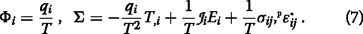Heat flux, q i , and stress, σ ij , will be defined in the next section. The plastic strain p ε ij comes from the small deformation plasticity, where the the total strain is decomposed additively into a reversible as well as irreversible (plastic) part

$$\varepsilon_{ij} = \,^{\text{r}}\!\varepsilon_{ij} + \,^{\text{p}}\!\varepsilon_{ij} \ .$$
(8)

By assuming small strains we can use the linear strain measure:

$$\varepsilon_{ij} = \frac{1}{2} \left(u_{i,j} + u_{j,i} \right) \,$$
(9)

where u i denotes the displacement field to be computed. Definition of the plastic strain will be given in the next section. Now we have found out the governing equation for the temperature,

$$\rho \frac{\partial \eta}{\partial t} + \left(\frac{q_{i}}{T} \right)_{,i} = \Sigma \ .$$
(10)

Initially the temperature is set at the so-called reference temperature of 300 K. Any deviation from the reference temperature induces a stress, which will be implemented via constitutive equations in the next section.

Induced stress causes a deformation. We search for displacements leading to that deformation. The stress, σ ji , is the momentum flux in the balance of linear momentum: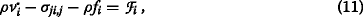where the specific body force f i is given—gravitational acceleration is a specific body force—and the production term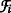defines the interaction with the electromagnetic forces. For the application that we want to study, the gravitational forces have a negligible effect, so we simplify the system by setting f i =0. For unpolarized systems, the production term is the LORENTZ force density: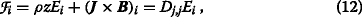since we have assumed that the magnetic flux vanishes, B i =0. The displacement has to fulfill the governing equation:

$$\rho \frac{\partial^{2} u_{i}}{\partial t^{2}} - \sigma_{ji,j} - D_{j,j} E_{i} = 0 \ .$$
(13)

We can compute ϕ, T, and u i from Eqs. (4), (8), (9), respectively, after having defined D i ,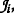, q i , η, σ ij , $$^{\text {p}}\!\varepsilon ^{{\bullet }}_{ij}$$ by means of ϕ, u i , T.

### Constitutive equations

We aim at defining the charge potential D i , the electric current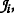, the heat flux q i , the specific entropy η, the stress σ ij , and the rate of plastic strain $$^{\text {p}}\!\varepsilon ^{{\bullet }}_{ij}$$. They are called constitutive or material equations closing the governing equations leading to partial differential equations of the electric potential ϕ, the displacement u i , and the temperature T.

The necessary connection for the charge potential is given by the so-called MAXWELL–LORENTZ aether relation:

$$D_{i} = \varepsilon_{0} E_{i} \,$$
(14)

with the universal constant ε 0=8.85·10−12 C/(V m). For the electric current we use OHM’s law:where the electrical conductivity, ς, is a material dependent parameter. For the heat flux we use FOURIER’s law:

$$\eta = c \ln\left(\frac{T}{T_{\text{ref.}}}\right) + \frac{1}{\rho} \alpha_{ij} \sigma_{ij} \,$$
(16)

with the material parameter κ called the thermal conductivity. The material parameters may depend on the temperature as well as electric field. Usually they are given as constants since such measurements are challenging. In order to define stress and entropy, we restrict the materials being simple such that their material parameters are constants. Then we can acquire for the entropy

$$\eta = c \ln\left(\frac{T}{T_{\text{ref.}}}\right) + \frac{1}{\rho} \alpha_{ij} \sigma_{ij} \,$$
(17)

and for the stress HOOKE’s law with DUHAMEL–NEUMANN extension:

$$\sigma_{ij} = C_{ijkl} \left(\varepsilon_{kl} - \,^{\text{p}}\!\varepsilon_{kl} - \,^{\text{th}}\!\varepsilon_{kl} \right) \,$$
(18)

$$\,^{\text{th}}\!\varepsilon_{ij} = \alpha_{ij} \left(T-T_{\text{ref.}} \right) \ .$$
(19)

The heat capacity c, coefficients of thermal expansion α ij , components of stiffness tensor C ijkl are assumed to be constant, otherwise the above material equations are not valid, for a thermodynamical derivation of all aforementioned constitutive equations, see (Abali 2016, Sect. 3.3).

In time the solution will be in a discrete fashion, where Δ t represents the time step. In order to calculate current (unknown) plastic strain, p ε ij , by using the (known) plastic strain from the last time step, $$\,^{\text {p}}\!\varepsilon ^{0}_{ij}$$, incrementally,

$$\,^{\text{p}}\!\varepsilon_{ij} = \,^{\text{p}}\!\varepsilon^{0}_{ij} + \Delta t \,^{\text{p}}\!\varepsilon^{{\bullet}}_{ij} \,$$
(20)

we use PRANDTL–REUSS theory with kinematic hardening

\begin{aligned} \,^{\text{p}}\!\varepsilon^{{\bullet}}_{mn} = \langle\gamma\rangle \frac{\left(\sigma^{0}_{|ij|}-\beta^{0}_{ij}\right)C_{ijkl}\left(\varepsilon_{kl}^{{\bullet}} - \,^{\text{th}}\!\varepsilon^{{\bullet}}_{kl}\right)}{\frac49 h \sigma_{\mathrm{Y}}^{2} + \left(\sigma^{0}_{|ij|} \,-\, \beta^{0}_{ij}\right)C_{ijkl}\left(\sigma^{0}_{|kl|} \,-\, \beta^{0}_{kl} \right)} \left(\!\sigma^{0}_{|mn|} \,-\, \beta^{0}_{mn}\right), \end{aligned}
(21)

where the material parameters h and σ Y are determined from a uniaxial tensile testing. The yield stress σ Y represents the threshold for plastic deformation. The slope of stress versus plastic strain is given by h. The so-called MACAULAY brackets as in 〈γ〉 defines a conditional parameter as being 1 or 0 depending on the VONMISES equivalent stress, σ eq, defined by the deviatoric stress, σ |ij|, as follows

$$\sigma_{\text{eq}} = \sqrt{\frac{2}{3} \sigma_{|ij|} \sigma_{|ij|}} \, \ \ \sigma_{|ij|} = \sigma_{ij} - \frac{1}{3} \sigma_{kk} \delta_{ij} \,$$
(22)

such that it becomes

$$\langle \gamma \rangle = \left\{ \begin{array}{ll} 1 & \text{if}~\sigma_{\text{eq}} \geq \sigma_{\mathrm{Y}} \\ 0 & \text{otherwise} \end{array} \right. \ .$$
(23)

The so-called back stress, β ij , evolves with the plastic stress, again incrementally,

$$\beta_{ij} = \beta^{0}_{ij} + \Delta t \beta^{{\bullet}}_{ij} \, \ \ \beta^{{\bullet}}_{ij} = \bar c \,^{\text{p}}\!\varepsilon^{{\bullet}}_{ij} \,$$
(24)

where we are going to choose $$\bar c=2h/3$$ in the simulations. A circuit board consist of copper traces and via embedded in a composite material. Since we want to detect the failure in the copper, we model the copper deforming elasto-plastically. Copper is a cubic material. In a circuit board copper has the thickness of 20–40 μm whereas its grain size is only 0.5 μm, see (Song et al. 2013). Hence we may assume that a polycristalline structure is present and the expected materials response is isotropic in this geometric scale. As a consequence of miniaturization this assumption may be critical in the near future. Hence we implement herein copper as a cubic material. For presenting the difference between isotropic and cubic materials, consider an isotropic material with the following material parameter tensors

$$C_{ijkl} = \lambda \delta_{ij} \delta_{kl} + \mu \left(\delta_{ik} \delta_{jl} + \delta_{il} \delta_{jk} \right) \, \ \ \alpha_{ij} = \alpha \delta_{ij} \,$$
(25)

where the LAME constants, λ, μ, and the thermal expansion constant α are the necessary material parameters. The parameters, λ, μ, read from the engineering constants, E, ν, G, which can be measured directly:

$${}\lambda = \frac{E\nu}{(1+\nu)(1-2\nu)} = \frac{2G\nu}{(1-2\nu)} \, \ \ \mu = \frac{E}{2(1+\nu)} = G \ .$$
(26)

YOUNG’s modulus, E, POISSON’s ratio, ν, and shear modulus, G, are coupled for isotropic materials as follows

$$G= \frac{E}{2(1+\nu)} \ .$$
(27)

In the case of a cubic material the latter relation fails to hold such that the material possesses three independent parameters, namely E, G, and ν need to be measured independently. We can write the stiffness tensor in a matrix notation

$$C_{IJ}=\left(\begin{array}{cccccc} C_{1111} & C_{1122} & C_{1133} & C_{1123} & C_{1113} & C_{1112} \\ C_{2211} & C_{2222} & C_{2233} & C_{2223} & C_{2213} & C_{2212} \\ C_{3311} & C_{3322} & C_{3333} & C_{3323} & C_{3313} & C_{3312} \\ C_{2311} & C_{2322} & C_{2333} & C_{2323} & C_{2313} & C_{2312} \\ C_{1311} & C_{1322} & C_{1333} & C_{1323} & C_{1313} & C_{1312} \\ C_{1211} & C_{1222} & C_{1233} & C_{1223} & C_{1213} & C_{1212} \end{array}\right) \,$$
(28)

called the VOIGT notation and calculate it as the inverse of the compliance matrix,

$$C_{IJ}=\left(S_{JI}\right)^{-1} \, \ \ S_{IJ} = \left(\begin{array}{cccccc} \frac{1}{E} & -\frac{\nu}{E} & -\frac{\nu}{E} & 0 & 0 & 0 \\ & \frac{1}{E} &-\frac{\nu}{E} & 0 & 0 & 0 \\ & & \frac{1}{E} & 0 & 0 & 0 \\ & & & \frac{1}{G} & 0 & 0 \\ & \text{sym.}& & & \frac{1}{G} & 0 \\ & & & & & \frac{1}{G} \end{array}\right) \ .$$
(29)

Analogously for the coefficients of thermal expansion

$$\alpha_{ij} = \left(\begin{array}{ccc} \alpha_{x} & 0 & 0 \\ & \alpha_{y} & 0 \\ \text{sym.} & & \alpha_{z} \end{array}\right) \,$$
(30)

we need to determine three independent coefficients for a cubic material. Necessary values for copper are taken from (Ledbetter and Naimon 1974, Table 10), (Deutsches Kupferinstitut 2014; Srikanth et al. 2007) as follows

$${} \begin{array}{cc} C_{IJ}^{\text{Cu}} = \left(\begin{array}{cccccc} 169.1 & 122.2 & 122.2 & 0 & 0 & 0 \\ & 169.1 & 122.2 & 0 & 0 & 0 \\ & & 169.1 & 0 & 0 & 0 \\ & & & 75.42 & 0 & 0 \\ & \text{sym.} & & & 75.42 & 0 \\ & & & & & 75.42 \end{array}\right)\! \cdot \! 10^{9}\,\text{Pa} \, \\ \alpha_{ij}^{\text{Cu}} = \left(\begin{array}{ccc} 17 & 0 & 0 \\ 0 & 17 & 0 \\ 0 & 0 & 17 \end{array}\right) \cdot 10^{-6} \,\mathrm{K}^{-1} \, \\ \sigma_{\mathrm{Y}}^{\text{Cu}} = 100\cdot 10^{6}\,\text{Pa} \, \ \ h^{\text{Cu}} = 615\cdot 10^{6}\,\text{Pa} \, \\ \rho^{\text{Cu}} = 8.94\cdot 10^{3} \,\text{kg/m}^{3} \, \\ \hspace{8pt} c^{\text{Cu}} = 390 \,\text{J/(kg K)} \, \ \ \kappa^{\text{Cu}} =385 \,\text{W/(K\,m)} \, \\ \varsigma^{\text{Cu}} = 5.8 \cdot 10^{7} \,\text{S/m} \ . \end{array}$$
(31)

The composite material for the board is a fiber-reinforced laminate structure. Fibers are placed orthogonal in a woven structure such that the board material is orthotropic. For an orthotropic material, the compliance matrix in the VOIGT notation reads

$$S_{IJ}^{\text{orth.}} = \left(\begin{array}{cccccc} \frac{1}{E_{x}} & -\frac{\nu_{xy}}{E_{y}} & -\frac{\nu_{xz}}{E_{z}} & 0 & 0 & 0 \\ & \frac{1}{E_{y}} &-\frac{\nu_{yz}}{E_{z}} & 0 & 0 & 0 \\ & & \frac{1}{E_{z}} & 0 & 0 & 0 \\ & & & \frac{1}{G_{yz}} & 0 & 0 \\ & \text{sym.}& & & \frac{1}{G_{zx}} & 0 \\ & & & & & \frac{1}{G_{xy}} \end{array}\right) \ .$$
(32)

All of 9 parameters need to be measured independently. Such a measurement is cumbersome. Instead, we can calculate the so-called homogenized parameters for the composite material. Consider different unidirectional plies stacked upon each other in such a way that we obtain an orthotropic material. In each unidirectional ply the material parameters can be calculated as a “weighted sum.” A ply consists of fiber and matrix—parameters of fiber and matrix are easier to obtain separately. Therefore, first we determine the materials data of each unidirectional ply. Secondly, we sum the properties by considering a particular orientation leading to the orthotropic board.

A unidirectional ply is transverse-isotropic. In order to identify material parameters, we choose a coordinate system, (x 1,x 2,x 3), where the first direction, x 1, is along the fibers in the ply. With respect to this so-called local coordinate system, we obtain the following compliance matrix in the VOIGT notation:

$$S_{IJ}^{\text{ply}} = \left(\begin{array}{cccccc} \frac{1}{E_{11}} & -\frac{\nu_{21}}{E_{11}} & -\frac{\nu_{21}}{E_{11}} & 0 & 0 & 0 \\ & \frac{1}{E_{22}} &-\frac{\nu_{23}}{E_{22}} & 0 & 0 & 0 \\ & & \frac{1}{E_{22}} & 0 & 0 & 0 \\ & & & \frac{2\left(1+\nu_{23}\right)}{E_{22}} & 0 & 0 \\ & \text{sym.}& & & \frac{1}{G_{12}} & 0 \\ & & & & & \frac{1}{G_{12}} \end{array}\right) \ .$$
(33)

These 5 parameters, E 11, E 22, ν 21, ν 23, and G 12 can be calculated from the parameters of matrix and fiber by using micromechanical rules, see (Schürmann 2005, §8). These rules are simple models based on the linear elasticity. The most important assumption is that matrix and fiber be connected perfectly, in other words, no voids or cracks are existing such that the length change of matrix and fiber are identical. Then we can combine the materials data of fiber and matrix; and we can calculate from them the parameters in a ply consisting of φ–fiber and (1−φ)–matrix as follows

\begin{aligned} \begin{array}{cc} E_{11} &= \varphi E_{11}^{\text{f.}} + (1-\varphi) E_{11}^{\text{m.}} \, \ \ E_{22} = \frac{E_{22}^{\text{m.}} E_{22}^{\text{f.}}}{\varphi E_{22}^{\text{m.}} + (1-\varphi) E_{22}^{\text{f.}}} \, \\ & \nu_{21} = \varphi \nu_{21}^{\text{f.}} + (1-\varphi) \nu_{21}^{\text{m.}} \, \\ \nu_{23} &= \varphi \nu_{23}^{\text{f.}} + (1-\varphi) \nu_{23}^{\text{m.}} \left(\frac{1+\nu_{23}^{\text{m.}}-\nu_{21}^{\text{f.}} \frac{E_{11}^{\text{m.}}}{E_{11}^{\text{f.}}}}{1-\left(\nu_{23}^{\text{m.}}\right)^{2} + \nu_{23}^{\text{m.}} \nu_{21}^{\text{f.}} \frac{E_{11}^{\text{m.}}}{E_{11}^{\text{f.}}}}\right) \, \\ & {G}_{12} = \frac{G_{12}^{\text{m.}} G_{12}^{\text{f.}}}{\varphi G_{12}^{\text{m.}} + (1-\varphi)G_{12}^{\text{f.}}} \, \end{array} \end{aligned}
(34)

and

\begin{aligned} \begin{array}{cc} \alpha_{11} = \frac{(1-\varphi) \alpha_{11}^{\text{m.}} E_{11}^{\text{m.}} + \varphi \alpha_{11}^{\text{f.}} E_{11}^{\text{f.}} }{ (1-\varphi)E_{11}^{\text{m.}} + \varphi E_{11}^{\text{f.}}} \, \\ \alpha_{22} = \varphi \alpha_{22}^{\text{f.}} \,+\, (1 \,-\, \varphi) \alpha_{22}^{\text{m.}} \, \ \ \alpha_{33} = \varphi \alpha_{33}^{\text{f.}} \,+\, (1 \,-\, \varphi) \alpha_{33}^{\text{m.}} \ . \end{array} \end{aligned}
(35)

The upper (·)m. and (·)f. denote the materials data of matrix and fiber, respectively. The materials data for s-glass, e-glass, and aramid are taken from (JPS Industries Inc. Company JPS Composite Materials 2017; Suter Kunststoffe AG 2017). The data of the epoxy matrix are found in (Soden et al. 1998). By using Eq. (29), parameters in Eq. (28) are calculated. All used and calculated parameters are compiled in Table 1. After having determined the parameters for a unidirectional ply, we can simply construct a laminate of several plies by stacking them orthogonally. The result is an orthotropic material. Owing to the linear constitutive equations, we can superpose each ply’s material tensors as transformed to the global coordinate system. All necessary materials data are compiled in Table 2. In addition to the aforementioned materials parameter, we use for laminate the following data:

\begin{aligned} \rho^{\text{lam.}} &= 2500\,{\text{kg/m}^{3}} \, \ \ c^{\text{lam.}} = 800\,\text{J/(kg\,K)} \, \\ \kappa^{\text{lam.}} &= 1.3\,\text{W/(m\,K)} \, \ \ \varsigma^{\text{lam.}} = 0 \ . \end{aligned}
(36)

### Weak form

The primitive variables, ϕ, u i , T, are continuous functions in space and time. We want to compute them by satisfying Eqs. (4), (9), (8) augmented by the constitutive equations introduced in the last section. We will approximate space by means of finite element method (FEM) and time by using finite difference method (FDM). Time discretization is quite intuitive, as a list of subsequent time steps, whereas for simplicity in programming we choose identical time steps

$$t = \{ 0, \Delta t, 2 \Delta t, \dots \} \ .$$
(37)

Instead of a partial time derivative, we write the following difference equations:

$$\frac{\partial (\cdot)}{\partial t} = \frac{(\cdot) - (\cdot)^{0}}{\Delta t} \, \ \ \frac{\partial^{2}(\cdot)}{\partial t^{2}} = \frac{(\cdot) - 2(\cdot)^{0} + (\cdot)^{00}}{\Delta t \Delta t} \,$$
(38)

where (·)0 and (·)00 indicate the computed values from the last and second last time steps, respectively. In order to approximate the functions in a discretized space, we multiply the governing equations by appropriate test functions and obtain a variational form for each primitive variable,

{{}{\begin{aligned} \mathrm{F}_{\phi} &= \int_{\Omega^{e}} \left(\frac{D_{i,i}-D_{i,i}^{0}}{\Delta t} + J_{i,i} \right) \updelta \phi \,\,\mathrm{d} V = 0 \, \\ \mathrm{F}_{\boldsymbol{u}} &= \int_{\Omega^{e}} \left(\rho \frac{u_{i} -2u_{i}^{0} +u_{i}^{00}}{\Delta t \Delta t} - \sigma_{ji,j} - D_{j,j} E_{i} \right) \updelta u_{i} \,\,\mathrm{d} V = 0 \,\\ \mathrm{F}_{T} &= \int_{\Omega^{e}} \left(\rho \frac{\eta-\eta^{0}}{\Delta t} + \Phi_{i,i} - \Sigma \right) \updelta T \,\,\mathrm{d} V = 0 \, \end{aligned}}}
(39)

integrated over a finite element Ω e. The forms F ϕ and F T are in the unit of power, whereas F u is in the unit of energy. By multiplying F ϕ and F T by Δ t, we obtain all forms in the same unit. The following terms: D i , J i , σ ji , Φ i consist of (space) derivatives of primitive variables. In the variational forms another derivative is addressed. Hence, the primitive variables have to be (at least) two times differentiable. This condition can be weakened by integrating by parts such that one of the derivatives is shifted to the corresponding test function, as follows

\begin{aligned} \mathrm{F}_{\phi} &= - \int_{\Omega^{e}} \left(D_{i}-D_{i}^{0} + \Delta t J_{i} \right) \updelta \phi_{,i}\,\,\mathrm{d} V \\ &\quad + \int_{\partial\Omega^{e}} \left(D_{i} - D_{i}^{0} + \Delta t J_{i} \right) \updelta \phi N_{i} \,\,\mathrm{d} A \, \\ \mathrm{F}_{\boldsymbol{u}} &= \int_{\Omega^{e}} \left(\! \rho \frac{u_{i} - 2 u_{i}^{0} +u_{i}^{00}}{\Delta t \Delta t} \updelta u_{i} + \sigma_{ji} \updelta u_{i,j} \right. \\ &\quad \left. - D_{j,j} E_{i} \updelta u_{i} {\vphantom{\int_{\partial\Omega^{e}}}}\right) \mathrm{d} V - \int_{\partial\Omega^{e}} \sigma_{ji} \updelta u_{i} N_{j} \,\,\mathrm{d} A, \\ \mathrm{F}_{T} &= \int_{\Omega^{e}} \left(\rho \left(\eta-\eta^{0}\right) \updelta T - \Delta t \Phi_{i} \updelta T_{,i} \right. \\ &\quad \left. - \Delta t \Sigma \updelta T \right) \mathrm{d} V + \int_{\partial\Omega^{e}} \Delta t \Phi_{i} \updelta T N_{i} \,\,\mathrm{d} A \, \end{aligned}
(40)

with N i being the plane normal pointing outward from Ω e. The latter integral forms are called the weak forms. The whole computational domain, Ω, consists of two different materials, each material is divided by finite elements satisfying F=0 with

$$\mathrm{F} = \mathrm{F}_{\phi} + \mathrm{F}_{\boldsymbol{u}} + \mathrm{F}_{T} \ .$$
(41)

We can assembly by summing over all elements. An element with its plane normal N i and its adjacent element with its opposing plane normal eliminate the boundary terms within a material. All primitive variables are continuous. Over the interface, Ω I, between different materials, there may occur jumps since the material parameters have different values. The weak forms read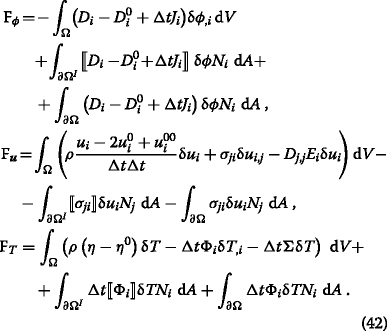On the interface, i.e., between two different materials, since ϕ is continuous, D i is continuous, too. No electric current is allowed along the normal direction, since copper is surrounded by the insulating board or air. According NEWTON’s lemma—action is equal to reaction—we expect that traction vectors t i =N j σ ji are also continuous. On the boundary, Ω, the traction vector $$\hat t_{i}$$ is given. In our example, we have free surfaces such that $$\hat t_{i}=0$$ on Ω or clamped faces where the displacement is given (as zero). On boundaries where the solution is given, we apply a DIRICHLET boundary condition and the test function vanishes. Temperature at the boundary can be modeled by using mixed boundary condition such that a deviation from the reference temperature causes a heat flux, $$q_{i} N_{i} = \bar h \left (T-T_{\text {ref.}}\right)$$, depending on the convective heat transfer coefficient $$\bar h$$ in J/(s m2 K). Finally, we acquire the weak form to be implemented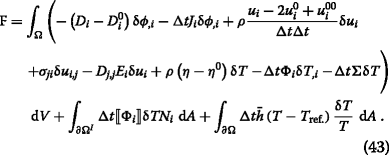We exploit the open-source packages developed under the FEniCS project and solve the coupled and nonlinear weak form for the simulations demonstrated in the next sections.

Under a cyclic loading, copper traces and via deform plastically and material fails after a number of cycles, N f.. Since plastic deformation is irreversible, in each cycle, plastic deformation accumulates. By means of computation we can determine the accumulated plastic strain in one cycle and use this as a measure of lifetime. The plastic strain rate, $$\,^{\text {p}}\!\varepsilon _{ij}^{{\bullet }}$$, is deviatoric, thus, the equivalent strain rate reads

$$\,^{\text{p}}\!\varepsilon_{\text{eq.}}^{{\bullet}} = \sqrt{\frac32 \,^{\text{p}}\!\varepsilon_{ij}^{{\bullet}} \,^{\text{p}}\!\varepsilon_{ij}^{{\bullet}} } \ .$$
(44)

The plastic strain accumulates in a cycle with the latter rate of equivalent strain,

$$\,^{\text{p}}\!\varepsilon_{\text{acc.}} = \int_{\text{cycle}} \,^{\text{p}}\!\varepsilon_{\text{eq.}}^{{\bullet}} \mathrm{d} t \ .$$
(45)

This accumulated strain is a distribution in the copper wire. Its mean value can be determined by averaging over a chosen volume V

$$\left\langle \,^{\text{p}}\!\varepsilon \right\rangle = \frac{\int_{V} \,^{\text{p}}\!\varepsilon_{\text{acc.}} \mathrm{d} V}{\int_{V} \mathrm{d} V}$$
(46)

This measure for a lifetime prediction, 〈 p ε〉, is computed by using the aforementioned approach. In order to establish a connection between the measure, 〈 p ε〉, and the number of cycles to failure, N f., there are various suggestions. They are mostly empirical such as computations and experiments need to be conducted and fitted. A theoretical analysis in (Manson 1968) provides the following relation

$$\langle \,^{\text{p}}\!\varepsilon \rangle = D^{0.6} N_{\text{f.}}^{-0.6} \,$$
(47)

for metallic compounds such as copper used in traces and via. The material specific constant, D, reads from the reduction of cross section, R, in a tensile test,

$$D = \ln\left(\frac{100}{100-R}\right) \ .$$
(48)

Cross section reduction, R, is in percentage and we take it as R=60 for the electrodeposited copper material, see (Valiev et al. 2002). It is important to recall that the parameter D can be obtained from a tensile testing. By employing computation we obtain 〈 p ε〉 and estimate the lifetime

$$N_{\text{f.}} = D \langle \,^{\text{p}}\!\varepsilon \rangle^{-5/3} \ .$$
(49)

This lifetime estimation is for the case of an accelerated test. It is challenging to determine a specific amount of months or years for the underlying electronic device. For comparing several designs, it is a helpful measure. A design with a longer lifetime is expected to be chosen from the point of mechanics.

## Results and discussion

For analyzing the multiphysics and estimating the number of failure on a simplified unpopulated—so-called bare-board—we choose realistic geometric dimensions used in the industry. As shown in Fig. 2, the CAD geometry is prepared and preprocessed in Salome v7.5 (Salome 2017) by using NETGEN algorithms (Schöberl 1997). We have chosen a board of dimensions 10×10×0.8 mm. Although all material parameters are given in SI units, we have converted them into mm, Mg (tonne), s, mA, K for the simulation such that the geometry is captured accurately (up to the machine precision) in mm. The conducting wire is called trace and via, both from the same material, namely 5N copper. We use a standard 1 oz. copper modeled with 35 μm of thickness. Starting from the back side of the board, a trace along x-axis is placed on top of the board. The trace has a width of 300 μm and is connected by the annular ring (pad) of 500 μm radius to the thru hole via. Trace and annular ring are produced by masking and etching. Actually the profile of the trace and ring is trapezoidal due to the etching process; however, we model it as rectangular. After ring and trace are plated, a hole with 200 μm radius is drilled and the via is electroplated with a given thickness. Herein we model the wall thickness of the via also as 35 μm. There are no standards for this thickness and the simulation results would be different by varying this thickness. Via connects the trace on top to the trace on bottom that runs until the front side of the board. Especially around traces and via, several refinements of the mesh are applied; the final finite element mesh can be seen in Fig. 3.

We present simulations of a possible measurement. All boundary conditions are selected as it would be the case in reality. Board is usually fastened by bolts on four holes near to the edges. In order to hold the board on four edges, we model chamfers on the edges and hold on these four chamfer faces as being clamped in all directions. We simply set the deformation zero as a DIRICHLET boundary condition. The conducting copper is driven by an electric potential difference. At the endings of traces on back and front faces, the electric potential is set as a DIRICHLET boundary condition. One end is grounded and the other is given harmonically,

$$\hat\phi = \phi_{\text{amp.}} \sin(2\uppi \nu t) \ .$$
(50)

For all simulations we have selected the period of 10 s leading to the frequency ν=0.1 Hz and an amplitude as ϕ amp.=0.2 V in order to reach high enough temperatures leading to significant plastic strains. Since we have only a highly conductive copper wire, even such a small potential difference lead to high electric currents and dissipated heat, JOULE’s loss. This setting is also configured in an accelerated fatigue test; but in an electronic device such conditions are not valid. Normally, there is a component like a resistor or capacitor connected to the circuit such that the electrical conductivity is lowered, leading to a smaller current, thus, a lower temperature increase. The computational model mimics a possible accelerated active fatigue experiment. In order to simulate an existing test; geometry and boundary conditions need to be accurately determined and applied.

The weak form in Eq. (43) is nonlinear. By using FEniCS packages the linearization is handled automatically at the level of the partial differential level, before the assembly. Solution is searched by a standard NEWTON–RAPHSON algorithm after assembly operation. Every time step lasts approximately 8 min on one (3 min on two) Intel Xeon Processors (i7-2600) running on Ubuntu 16.04.

In order to comprehend the complicated multiphysics bearing electro-thermo-mechanical coupling, we present several results on one board at the quarter of one period, t=2.5 s. The results with other laminates are qualitatively the same. The potential difference generates an electric current. It is in equal amount in ampere along the trace and via. The current density,, is the amount per cross section. Since we have chosen the trace as well as via of the same thickness and the circumference of the via is longer than the width of the trace, the current density is greater along the trace than through the via. The electric potential and current density can be seen in Fig. 4. Greater electric current density implies a greater JOULE’s heat,, directly responsible for the temperature increase. Hence, the temperature increase is more on the trace than on the via. The temperature distribution again at the quarter period can be depicted in Fig. 5. For another wall thickness, this result would be different.

It is of importance to recall that the temperature distribution is not homogeneous, which is indeed the case in reality. This fact is overseen in an accelerated fatigue test performed in an oven. Temperature is changed quickly in the chamber, as a consequence, a homogeneous temperature distribution emerges, since the board is thin and copper is a good conductor. In this configuration the damage occurs in the via. In an active test, however, we realize that the temperature distribution is heterogeneous that lead to another deformation mode in each cycle. In order to visualize the deformation, see Fig. 6. It is interesting to see that the middle part of the via is not moving; however, the variation of the displacement along the hole still induces a strain.

Approximately more than 20 K deviation from the reference temperature T ref.=300 K results stresses higher than the yield stress and plastic deformation starts accumulating. Simultaneously, heat escapes to the ambient, in the simulation we use the same convective heat transfer coefficients for the board as well as via, $$\bar h = 10$$ J/(s m2 K), modeling a relatively slow free convection. This parameter is very difficult to measure accurately such that we choose a value and use the same for all simulations.

Temperature is produced within the conductor and exchanged over its boundary at the same time. Change across the boundary is greater when the temperature on the boundary increases. However, since we model free convection, this rate is small compared to the heat production. Depending on the excitation frequency of the given electric potential and depending on the convective heat transfer coefficient, the temperature increases until the heat exchange rate and production are equal in their absolute values. This steady-state condition is difficult to reach in the simulation, at least for the first 5 cycles the steady-state is not reached, see Fig. 7. We realize that a real experiment with the aforementioned setting might be difficult since within one minute the melting temperature of the board would be reached. Either a forced convection (using a fan) or a resistor connected to the circuit decreases the temperature increase. Although the increase is high, the total difference between the maximum and minimum temperature remains approximately the same in every cycle.

The fatigue failure occurs mostly because of the plastic strain accumulation. At the end of the first cycle, see the equivalent plastic strains in Fig. 8. The heterogeneous temperature distribution and the presented deformation lead to high plastic strains in the trace as well as in the via. This result is different compared to a fatigue experiment in an oven, where the most of the plastic strain accumulates within the via. Herein, in an active testing, we observe especially at the middle height of the via higher values than within the trace. For a better comparison we determine the mean values in two different volumes: over the traces and over the via. The accumulation of the mean value of the plastic strain averaged over these two regions can be seen in Fig. 9. Due to the irreversible character, the plastic strain accumulates whenever the temperature is increasing and remains the same at the moment when the temperature is decreasing. In every cycle the amount of the newly accumulated plastic strain is compiled in Table 3. A steady-state cannot be reached before the temperature variation gets stabilized.

Results in all laminates are qualitatively similar. They do differ quantitatively in terms of the plastic strain. In order to compare different laminates and their effects to the fatigue behavior, we conduct three simulations and compute the mean accumulated plastic strain at the end of one cycle. Again the aforementioned two volumes are used for averaging. The choice of the averaging volume is somehow heuristic and challenging; however, for a comparison between three laminates, the choice fails to be relevant. The values are compiled in Table 4.

By considering the fatigue as the sole criterion, it is fair to claim that the laminate III—aramid reinforced epoxy composite material—performs better than glass fiber reinforced epoxy materials. Since the governing equations are coupled and nonlinear, such a conclusion is challenging to predict based on the material parameters. Laminate III has the highest thermal expansion coefficient along the plate thickness, so the mismatch between expansion coefficients is higher. Hence, it is intuitive to guess that it would lead to greater plastic strains. As we see from the deformation mode, the boundary conditions lead to a more shearing deformation that is the real reason of a plastic deformation. Expansion along the board thickness reduces shearing deformation leading to smaller plastic strains. Based on only one of the material parameters, we might prejudge the outcome differently than the observation by means of computations as presented herein. There are many coupling effects acting simultaneously, the only prediction shall be based on simulations with the least number of assumptions. Herein we present a robust method for computing an electro-thermo-mechanical system. In order to verify the code, we need to simulate existing experiments by correctly choosing boundary conditions as well as the geometry. According to the demonstrated comparison, a different choice for the laminate composition might increase the fatigue strength. There is a growing attention to find out variations of FR4 PCBs out of e-glass and epoxy. Stablcor Technology Inc. has patented its own PCF consisting of carbon fibers. Thermount is a registered trademark by DuPont and it uses aramid fibers.

Coupled computations is of importance to obtain a detailed investigation guiding toward newer insight into multiphysics. Herein we have neglected magnetic potential and thermoelectric effects. Often more assumptions are undertaken in order to simplify or decouple the governing equations leading to a fast simulation. With today’s technological possibilities, we can perform computations as presented herein by using a laptop. Hence, we can get a detailed understanding of the phenomenon and even suggest design changes. In order to enable a scientific exchange, we deliver our codes in (Abali 2011) to be used under the GNU Public license (GNU Public 2017).

## Conclusions

Using rational continuum mechanics, all necessary governing equations and constitutive equations are presented for an electro-thermo-mechanical system. We have directly attacked an application from electronics industry, namely a phenomenon called fatigue in copper vias. Accelerated experiments are generally conducted in an oven so the temperature is controlled globally. In the recent years, more sophisticated experiments started to emerge, where the electric potential is controlled that leads to a local heating. This multiphysics problem is challenging to compute numerically, since the governing equations are nonlinear and coupled. We have presented an approach for computing coupled and nonlinear governing equations by means of open-source packages and simulated the electro-thermo-mechanical system monolithically. The results seem to be promising, a verification with experimental results is left to future research.

## Abbreviations

FDM:

Finite difference method

FEM:

Finite element method

PCB:

Printed circuit board

## References

1. Abali, BE (2011) Technical University of Berlin, Institute of Mechanics, Chair of Continuums Mechanics and Material Theory, Computational Reality. http://www.lkm.tu-berlin.de/ComputationalReality/.

2. Abali, BE, Reich FA, Müller WH (2014a) Fatigue analysis of anisotropic copper-vias in a circuit board. GMM, Mikro-Nano-Integration. VDE Verlag, Berlin.

3. Abali, BE, Lofink P, Müller WH (2014b) Variation of plastic materials data of copper and its impact on the durability of Cu-via interconnects. In: Aschenbrenner R Schneider-Ramelow M (eds)Microelectronic Packaging in the 21st Century, 305–308.. Fraunhofer Verlag. Chap. 7.2.

4. Abali, BE (2016) Computational Reality, Solving Nonlinear and Coupled Problems in Continuum Mechanics. Advanced Structured Materials. Springer, Singapore.

5. Alnaes, MS, Mardal KA (2012). In: Logg A, Mardal K-A, Wells GN (eds)Automated solution of differential equations by the finite element method, the FEniCS book.. Springer. Chap. 15 Syfi and sfc: symbolic finite elements and form compilation.

6. Atli-Veltin, B, Ling H, Zhao S, Noijen S, Caers J, Weifeng L, Feng G, Yuming Y (2012) Thermo-mechanical investigation of the reliability of embedded components in pcbs during processing and under bending loading In: Thermal, Mechanical and Multi-Physics Simulation and Experiments in Microelectronics and Microsystems (EuroSimE), 2012 13th International Conference On, 1–4.. IEEE.

7. Deutsches Kupferinstitut (2014) Kupfer in der Elektrotechnik – Kabel und Leitungen. www.kupferinstitut.de.

8. FEniCS project (2017) Development of tools for automated scientific computing: 2001–2016. http://fenicsproject.org.

9. GNU Public (2017) Gnu general public license. http://www.gnu.org/copyleft/gpl.html.

10. JPS Industries Inc. Company JPS Composite Materials (2017). http://jpsglass.com/jps_databook.pdf.

11. Kpobie, W, Martiny M, Mercier S, Lechleiter F, Bodin L, des Etangs-Levallois AL, Brizoux M (2016) Thermo-mechanical simulation of pcb with embedded components. Microelectron Reliab 65: 108–130.

12. Ledbetter, H, Naimon E (1974) Elastic properties of metals and alloys. ii. copper. J Phys Chem Ref Data 3(4): 897–935.

13. Manson, S (1968) A simple procedure for estimating high-temperature low-cycle fatigue. Exp Mech 8(8): 349–355.

14. Ridout, S, Bailey C (2007) Review of methods to predict solder joint reliability under thermo-mechanical cycling. Fatigue Fract Eng Mater Struct 30(5): 400–412.

15. Roellig, M, Dudek R, Wiese S, Boehme B, Wunderle B, Wolter KJ, Michel B (2007) Fatigue analysis of miniaturized lead-free solder contacts based on a novel test concept. Microelectron Reliab 47(2): 187–195.

16. Salome (2017) The Open Source Integration Platform for Numerical Simulation. http://www.salome-platform.org.

17. Schürmann, H (2005) Konstruieren Mit Faser-Kunststoff-Verbunden. Springer, Berlin.

18. Schöberl, J (1997) NETGEN an advancing front 2d/3d-mesh generator based on abstract rules. Comput Vis Sci 1(1): 41–52.

19. Solomon, HD (1991) Predicting thermal and mechanical fatigue lives from isothermal low cycle data In: Solder Joint Reliability, 406–454.. Springer.

20. Song, JM, Wang DS, Yeh CH, Lu WC, Tsou YS, Lin SC (2013) Texture and temperature dependence on the mechanical characteristics of copper electrodeposits. Mater Sci Eng A 559: 655–664.

21. Soden, P, Hinton M, Kaddour A (1998) Lamina properties, lay-up configurations and loading conditions for a range of fibre-reinforced composite laminates. Compos Sci Technol 58(7): 1011–1022.

22. Srikanth, N, Premkumar J, Sivakumar M, Wong Y, Vath C (2007) Effect of wire purity on copper wire bonding In: Electronics Packaging Technology Conference, 2007. EPTC 2007. 9th, 755–759.. IEEE.

23. Suter Kunststoffe AG (2017). http://www.swiss-composite.ch/pdf/i-Werkstoffdaten.pdf.

24. Valiev, R, Alexandrov I, Zhu Y, Lowe T (2002) Paradox of strength and ductility in metals processed bysevere plastic deformation. J Mater Res 17(01): 5–8.

## Acknowledgements

This work was completed while B. E. Abali was supported by a grant from the Max Kade Foundation to the University of California, Berkeley.

### Funding

This work was completed while B. E. Abali was supported by a grant from the Max Kade Foundation to the University of California, Berkeley.

### Availability of data and materials

All codes used for simulations are publicly available in (Abali 2011) licensed under the GNU Public license (GNU Public 2017).

### Competing interests

The author declares that he/she has no competing interests.

### Publisher’s Note

Springer Nature remains neutral with regard to jurisdictional claims in published maps and institutional affiliations.

## Author information

Authors

### Corresponding author

Correspondence to B. Emek Abali.

## Rights and permissions